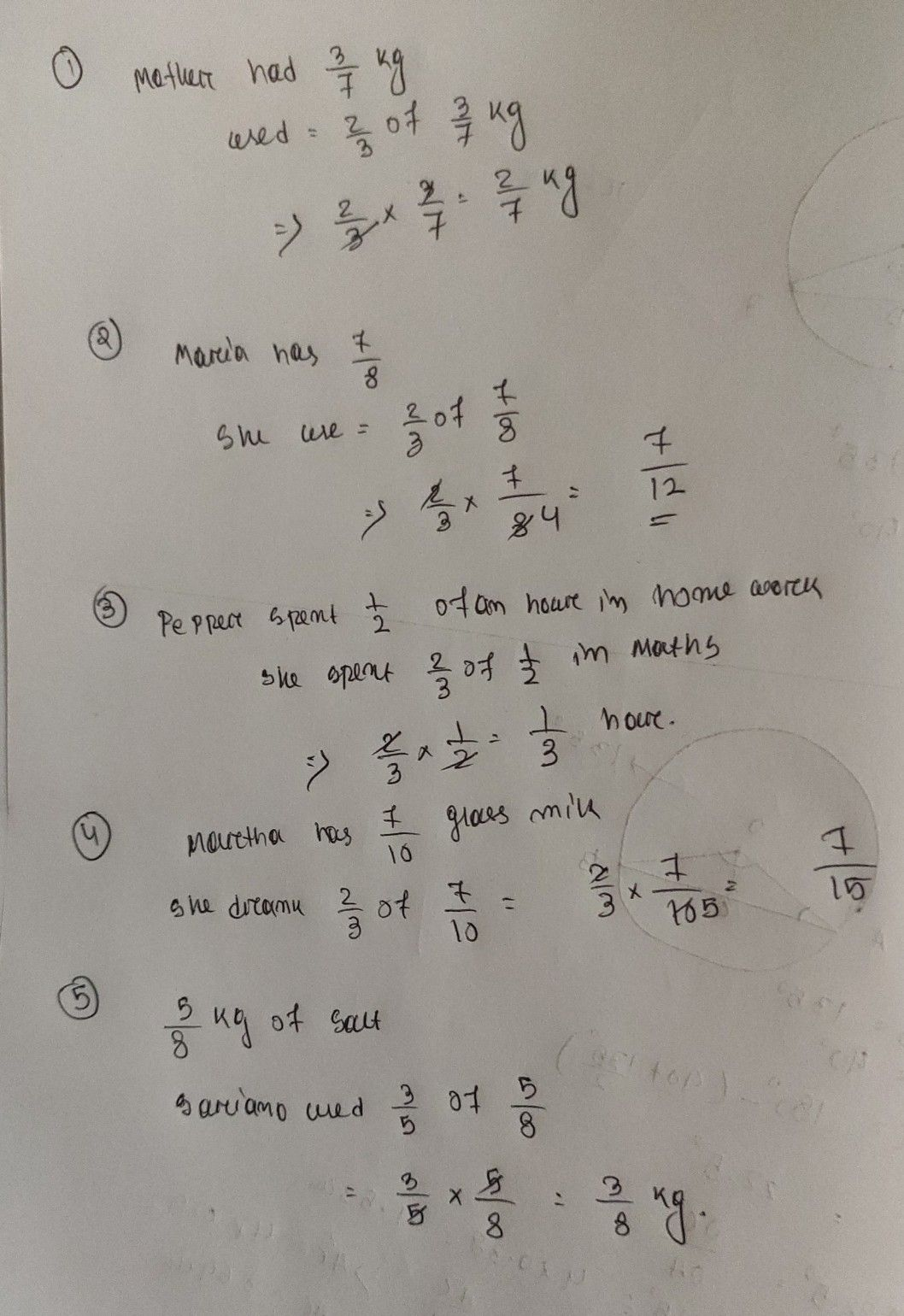Symbol
Problem1. $1other$ had kg of flour. She used of it to make pancakes, How much flour did she use to $mak6$ B. $Dircctions$ Solve the following $problcms$ $2.$ $Ma\pi ia$ has - tank of gasoline in her car. She estimates that she will $1sc\dfrac {2} {3}$ - of the gasoline to get $Pancakcs2$ kg $h0mc$ $Vha$ fraction of the tank of gasoline will she use? 2 of it doing Math. $Hox$ much time did 3. Pepper spent of an hour doing her homework. She $spcnt$ she spend doing her Math homework? 4. Martha has - 10 $glass$ of milk tea. She $drank\dfrac {2} {3}$ of it. How much $glass$ of milk tea did she drink? 5. $rhcrcarc\dfrac {5} {8}k$ $kailogTams$ of salt in the kitchen. Mrs. $sorian9$ used of the salt when she was preparing dinner. How much salt did she $usc7$
1st-6th grade
Other
Search count: 105
SolutionQanda teacher - Pranab